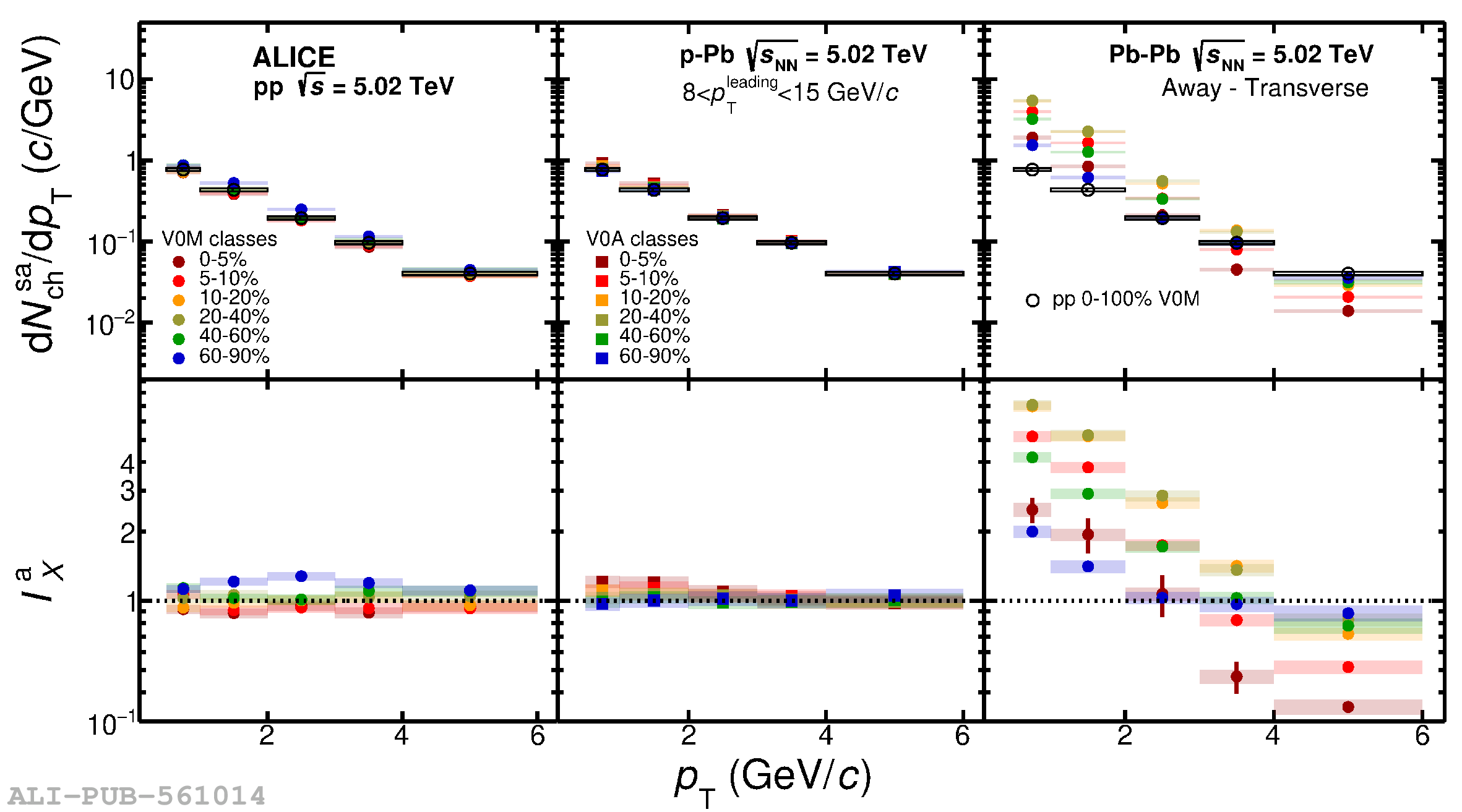# Figure 3

 Transverse momentum spectra of charged particles in Toward-Transverse, ${\rm{d}}N_{\rm{ch}}^{\rm{st}}/{\rm{d}}p_{\rm T}$ (top plot) and Away-Transverse, ${\rm{d}}N_{\rm{ch}}^{\rm{sa}}/{\rm{d}}p_{\rm T}$ (bottom plot) regions for different multiplicity classes in pp (left), p$-$Pb (middle) and Pb$-$Pb (right) collisions at $\sqrt{s_{\rm NN}}=5.02$ TeV. The $p_{\rm T}$ spectra are measured at mid pseudorapidity ($|\eta|<0.8$). The lower panels of both plots show the ratio to minimum bias pp collisions. The statistical and systematic uncertainties are shown by bars and boxes, respectively.